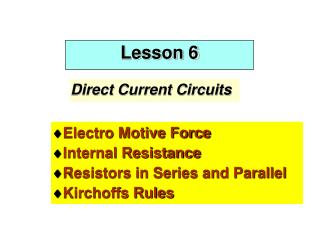DownloadDownload PresentationLesson 6

# Lesson 6

Télécharger la présentation## Lesson 6

- - - - - - - - - - - - - - - - - - - - - - - - - - - E N D - - - - - - - - - - - - - - - - - - - - - - - - - - -
##### Presentation Transcript

1. Lesson 6 Lesson 6 Direct Current Circuits • Electro Motive Force • Internal Resistance • Resistors in Series and Parallel • Kirchoffs Rules

2. Topics • RC Circuits • Charging • Discharging Capacitors • Electrical Instruments • Galvanometer • Ammeter • Voltmeter • Wheatstone Bridge • Potentiometer

3. EMF I Electro Motive Force (emf) Source of emf is any device that increases the potential energy of charges circulating in a circuit. Electric Potential increases by the emf Eas charge goes from negative to positive plate of battery.

4. EMF II EMF is Work Done per unit charge by electrical pump e dW = dQ

5. Charge Pump • Battery is a Charge Pump • Current flowing internally in battery feels a resistance this is an • Internal resistance, r • Flowing positive charges (current)experience drop of electric potential in resistor V=IR + - R

6. Internal Resistance - - - + + + Terminals • Terminal Potential Difference • V = E - Ir

7. Picture

8. Power and Internal Resistance

9. Combination of Resistors Combinations of Resistors • Parallel • same electric potential felt by each element • Series • electric potential felt by the combination is the sum of the potentials across each element

10. Series

11. Parallel

12. Kirchoffs Rules I Kirchoff’s Rules The sum of the currents entering a junction must equal the sum of the currents leaving Conservation of Charge

13. Kirchoffs Rules II The Sum of the Potential Differences around a closed circuit loop must be zero Conservation of Energy

14. Picture

15. RC circuits RC Circuits • Non Steady State • Non Equilibrium • Current varies with time

16. Picture

17. Charging I

18. Charging II q e - - = IR 0 C æ ö e d q dI 1 dq - - = = - - ç ÷ IR 0 0 R è ø dt C dt C dt dI I Û + = R 0 dt C dI 1 Þ = - dt I RC ò ò t I dI 1 Þ = - dt I RC I 0 0 æ ö t I t - ( ) Þ ç ÷ = - Û = ln I t I e RC 0 è ø I RC 0 e t - ( ) = RC I t e R

19. e Charging III t - ( ) = RC I t e R e t dq - = e RC dt R e t - Þ = RC dq e dt R ò ò q t e t - = dq e RC dt R 0 0 é ù é ù t t e - - e e ê ú ê ú ( ) RC RC Þ = - = - C q t 1 Q 1 ê ú ê ú ë û ë û

20. Time Constant

21. Discharging I Discharging +Q -Q q = IR from Kirchoff C dq = - I the rate of decrease of charge dt

22. Discharging II